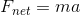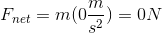# GED Science : Newton's Second Law

## Example Questions

### Example Question #1 : Motion And Mechanics

Which of the following best illustrates Newton's Second Law?

As a hammer hits a nail, the nail also exerts a force on the hammer

When two cymbals collide, some kinetic energy is converted into sound

A ball is rolled across a table and does not stop until it hits an area with friction

If an object is not accelerating, then the net force on it is zero

If an object is not accelerating, then the net force on it is zero

Explanation:

Newton's Second Law is best represented as an equation, in which the product of mass and acceleration is equal to net force:By this principle, if there is zero acceleration, then the force must also be zero.A non-zero acceleration must be present if there is a non-zero net force.